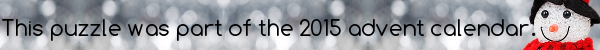mscroggs.co.uk
mscroggs.co.uksubscribe

# Puzzles

## What is the sum?

What is $$\displaystyle\frac{1}{\sqrt{1}+\sqrt{2}}+\frac{1}{\sqrt{2}+\sqrt{3}}+...+\frac{1}{\sqrt{15}+\sqrt{16}}$$?

## 19 December1 = 1/2 + 1/4 + 1/8 + 1/16 + 1/16. This is the sum of 5 unit fractions (the numerators are 1).
In how many different ways can 1 be written as the sum of 5 unit fractions? (the same fractions in a different order are considered the same sum.)

## Shooting hoops

Source: Alex Bolton
You spend an afternoon practising throwing a basketball through a hoop.
One hour into the afternoon, you have scored less than 75% of your shots. At the end of the afternoon, you have score more than 75% of your shots.
Is there a point in the afternoon when you had scored exactly 75% of your shots?

## Odd sums

What is $$\frac{1+3}{5+7}$$?
What is $$\frac{1+3+5}{7+9+11}$$?
What is $$\frac{1+3+5+7}{9+11+13+15}$$?
What is $$\frac{1+3+5+7+9}{11+13+15+17+19}$$?
What is $$\frac{\mathrm{sum\ of\ the\ first\ }n\mathrm{\ odd\ numbers}}{\mathrm{sum\ of\ the\ next\ }n\mathrm{\ odd\ numbers}}$$?

## Archive

Show me a random puzzle
▼ show ▼